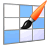# iRubric: Kindergarten - Counting and Comparing rubric

 find rubric
 (draft)           test run   apply to...   delete .rubinfoitem { padding:5px; white-space: nowrap; } Kindergarten - Counting and Comparing  Rubric Code: Q227BCX Draft Public Rubric Subject: Math   Type: Assessment   Grade Levels: K-5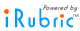Counting and Comparing Standards and Objectives Objective 1: The student will use procedural fluency and conceptual understanding to count, count forward from a given number, write numbers, count to answer how many using numbers. Students will score a 7 out of 8 on a rubric to prove proficiency. Objective 2: The student will use procedural fluency and conceptual understanding to compare two numbers and identify whether the numbers are greater than, less than, or equal to. Students will score a 3 out of 4 on a rubric to prove proficiency Procedural Fluency The student shows the ability to apply procedures accurately and efficiently. 1 pts Conceptual Understanding The students shows comprehension of mathematical concepts operations, and relations. 1 pts K.CC.1 Counting Count to 100 by ones, fives, and tens Procedural Fluency 1.) Students can count 34 thumbs and fingers and then circle the correct number of fingers and thumbs. Conceptual Understanding 2.) Students can look at the two counting sequences. Students will circle the first sequence of numbers and cross out the other. K.CC.2 Counting Count forward beginning from a given number within the known sequence (instead of having to begin at 1) Procedural Fluency 3.) Students can start with the number 33. Count up 10 and circle the number 43. Conceptual Understanding 4.) Students can start with 27 and count on 5. Students will then answer question. What number should be in the box with the X? Students will answer 33. K.CC.3 Counting Write numbers from 0 to 20. Represent a number of objects with a written numeral 0-20 (with 0 representing a count of no objects) Procedural Fluency 5.) Students can count the 15 flowers and write the number on the line. Conceptual Understanding K.CC.3 Counting Write numbers from 0 to 20. Represent a number of objects with a written numeral 0-20 (with 0 representing a count of no objects) Procedural Fluency 6.) Students can write numbers 9,13,14,16, on the lines. Conceptual Understanding K.CC.5 Counting Count to answer “how many?” questions about as many as 20 things arranged in a line, a rectangular array, or a circle, or as many as 10 things in a scattered configuration; given a number from 1–20, count out that many objects Procedural Fluency 7.) Students can count the set of 16 diamonds and write the number on the line. Conceptual Understanding 8.) Students can draw 12 circles in a circle. K.CC.6 Comparing Identify whether the number of objects in one group is greater than, less than, or equal to the number of objects in another group, e.g., by using matching and counting strategies Procedural Fluency 9.) Students can circle the group of cats. Conceptual Understanding 10.) Students can draw more triangles to make the 2 sets equal. K.CC.7 Comparing Compare two numbers between 0 to 10 presented as written numerals Procedural Fluency 11.) Students can circle the less than symbol. Conceptual Understanding 12.) Students can rewrite the problem to reflect a true statement.

Keywords:

Subjects:

Types:

You may also be interested in:

Do more with this rubric:Preview this rubric.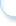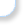Modify this rubric.Make a copy of this rubric and begin editing the copy.Show a printable version of this rubric.Add this rubric to multiple categories.Bookmark this rubric for future reference.Assess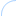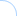This rubric is still in draft mode and cannot be scored. Please change the rubric status to ready to use.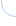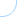ShareLink, embed, and showcase your rubrics on your website.Email this rubric to a friend.Discuss this rubric with other members.Do more with rubrics than ever imagined possible. Only with iRubrictm.